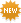We Are The Ones lyrics by Son Lux - original song full text. Official We Are The Ones lyrics, 2020 version | LyricsMode.comRequest & respond explanations
• Don't understand the meaning of the song?
• Highlight lyrics and request an explanation.
• Click on highlighted lyrics to explain.
Son Lux – We Are The Ones lyrics
[Intro: Vocal Ensemble]
Now, now, now, now
Now, now, now, now
Now, now, now, now
Now, now, now, now

[Verse 1: Ryan Lott]
Now, the wind
Now, a voice it carries
You know the one this time

[Verse 2: Ryan Lott]
Now, a breath
Now, a name I'm calling
Yours is the one this time

[Chorus 1: Vocal Ensemble]
We are the ones this time
We are the ones this time
We are the ones this time
We are the
We are the ones this time
We are the ones this time
We are the ones this time
We are the

[Verse 3: Ryan Lott]
Now, the wind
Now, a voice it carries
I know the one this time

[Verse 4: Ryan Lott & Vocal Ensemble]
Now, a breath (We are the ones)
Now, a name you're calling
Mine is the one this time (We are the ones this time)

[Chorus 1: Vocal Ensemble]
We are the ones this time
We are the ones this time
We are the ones this time
We are the
We are the ones this time
We are the ones this time
We are the ones this time
We are the

[Chorus 2: Vocal Ensemble]
Now, we are the ones this time
We are the ones this time
We are the ones this time
We are the
We are the ones this time
We are the ones this time
We are the ones this time
We are the

[Outro: Vocal Ensemble]
Now, now, now, now
Now, now, now, now
Now, now, now, now
Now, now, now

×

Lyrics taken from /lyrics/s/son_lux/we_are_the_ones.html

• Email
• Correct

## We Are The Onesmeanings

Know what this song is about? Does it mean anything special hidden between the lines to you? Share your meaning with community, make it interesting and valuable. Make sure you've read our simple .
U
Min 50 words
Good
Awesome!

U
Min 50 words